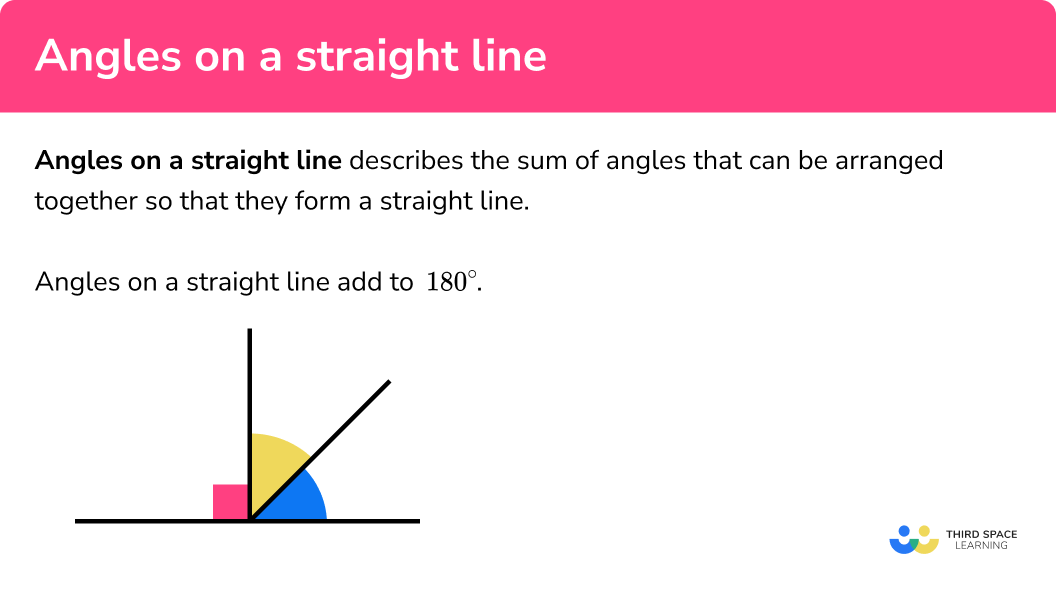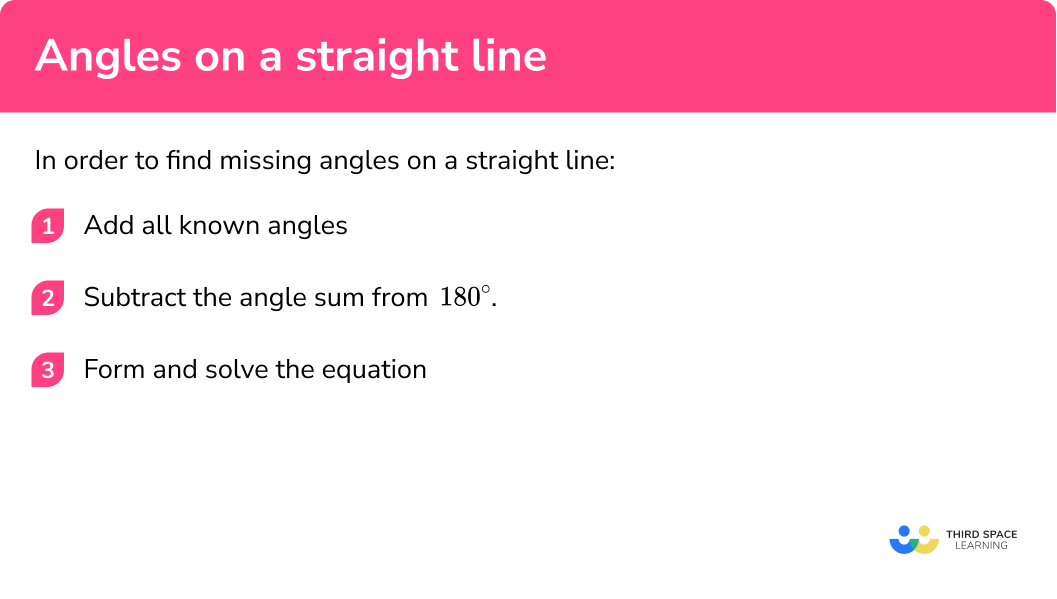# Angles On A Straight Line

Here we will learn about angles on a straight line, including the sum of angles on a straight line, how to find missing angles, and using these angle facts to generate equations and solve problems.

There are also angles on a straight line worksheets based on Edexcel, AQA and OCR exam questions, along with further guidance on where to go next if you’re still stuck.

## What are angles on a straight line?

Angles on a straight line relate to the sum of angles that can be arranged together so that they form a straight line.

Angles on a straight line add to 180°.

Let us look at this visually:

Let’s take the three angles of a, b, and c.

a is a right angle as it measures 90°, b = 38°, and c = 52°

If we move these three angles so that each vertex meets, we get an arrangement that looks like this:

These three angles create a straight line.

By adding together a = 90°, b = 38°, and c = 5° we can see the sum of the angles on a straight line is 180°.

We can also look at this in reverse by considering how many degrees it takes to do a full turn.

If you stand facing North, and turn to face East, you have turned 90° clockwise. A further turn of 90° clockwise will mean you would now be facing South. Repeating this turn twice more, you would first face West, and then back to North (the full turn). Half a turn is therefore 2 lots of 90° which is 180°

We can therefore state that the sum of angles on a straight line is equal to 180°. If we split any straight line into smaller angles, all of these angles would add to make 180°, the same as with a triangle.

Step by step guide: Angles in a triangle

Angles in a straight line are a problem solving tool for many geometric problems. These include: properties of shapes, circle theorems, angles in parallel lines, calculating angles in shapes (interior and exterior angles), trigonometry and more.

### What are angles on a straight line?## How to find missing angles on a straight line

In order to find missing angle on a straight line:

2. Subtract the angle sum from 180°.
3. Form and solve the equation.

### How to find missing angles on a straight line## Angles on a straight line examples

### Example 1: obtuse angles

AB is a straight line through O. Calculate the missing angle x.

$78^{\circ}$

2Subtract the angle sum from 180°.

$180-78=102^{\circ}$

$x=102^{\circ}$

### Example 2: acute angles

AB is a straight line through O. Calculate the missing angle x.

Subtract the angle sum from 180°.

### Example 3: right angles

AB is a straight line through O. Calculate the missing angle x.

Subtract the angle sum from 180°.

### Example 4: vertically opposite angles

AB and CD are straight lines. Calculate the value x.

Subtract the angle sum from 180°.

Form and solve the equation.

### Example 5: forming and solving equations

AB is a straight line through O. Calculate the size of all the angles that make up the line AB.

Form and solve the equation.

### Example 6: circles and tangents

AB is a tangent to the circle with centre C. The tangent intersects the circle at the point O on the circumference. Use this information to calculate the value of x.

Form and solve the equation.

### Common misconceptions

• The sum of angles on a straight line is equal to 360°

The angle sum is remembered incorrectly as 360°, rather than 180°. The sum of angles on a straight line is half of a full turn, which is 180°.

• Solving equations

There are many misconceptions around forming and solving equations. See the lesson on Solving Equations for further information.

Step by step guide: Solving equations

• Using a protractor

When you are asked to calculate a missing angle, a common error is to use a protractor to measure the angle. When using angle facts to determine angles, diagrams are deliberately not drawn to scale unless the angle is 90° or 180° as these are important angles to recognise. You should not use a protractor for this style of question.

Angles on a straight line is part of our series of lessons to support revision on angle rules. You may find it helpful to start with the main angle rules lesson for a summary of what to expect, or use the step by step guides below for further detail on individual topics. Other lessons in this series include:

### Practice angles on a straight line questions

1. AOB is a straight line. Calculate the size of angle x .95^{\circ}25^{\circ}115^{\circ}295^{\circ}180-65=115^{\circ}

2. AOB is a straight line. Calculate the size of angle x .238^{\circ}58^{\circ}32^{\circ}122^{\circ}180-122=58^{\circ}

3. Calculate the size of the angle 2x . Hence find the value of x .26^{\circ}52^{\circ}76^{\circ}116^{\circ}2x+90+38=180

2x+128=1804. AB and CD are straight lines. Calculate the size of angle BOD. Hence find the value of x .8^{\circ}40^{\circ}220^{\circ}12^{\circ}5x+140=1805. AOB is a straight line. By finding the value for x , calculate the size of each angle in the diagram below.x=35^{\circ}, COD=175^{\circ}, DOE=15^{\circ}, EOB=165^{\circ}x=12.5^{\circ}, COD=72.5^{\circ}, DOE=7.5^{\circ}, EOB=75^{\circ}x=11.1^{\circ}, COD=55.5^{\circ}, DOE=8.9^{\circ}, EOB=69.4^{\circ}x=2.05^{\circ}, COD=10.2^{\circ}, DOE=17.95^{\circ}, EOB=33.18^{\circ}The circle with centre C has a tangent at point O. Calculate the value of x correct to 2 decimal places.19.11^{\circ}10.59^{\circ}9.11^{\circ}5.29^{\circ}### Angles on a straight line GCSE questions

1. Given that the sum of exterior angles of any regular polygon is equal to 360^{\circ} , calculate the interior angle of a regular pentagon.

(2 marks)

360\div5=72^{\circ}

(1)

180-72=108^{\circ}

(1)

2.

(a) 3 straight lines intersect to form a triangle.Calculate the size of angle x .
(b) What type of triangle is ABC?

(4 marks)

a)

180-131=49

(1)

180-87=93

(1)

x=180-(93+49)=38^{\circ}

(1)

b)

A scalene triangle.

(1)

3. AB and CD are parallel lines. OE transects both lines.Calculate the value of x .

(3 marks)

4x+22.4=180

(1)

4x=157.6

(1)

x=39.4^{\circ}

(1)

4. AB is a straight line that is a tangent to the circle at point O. Show that OCD is a right angle triangle.(3 marks)

ODC=180-112=68^{\circ}

(1)

180-(68+22)=90^{\circ}

(1)

One angle in triangle OCD is equal to 90^{\circ} so it is a right angle triangle.

(1)

## Learning checklist

You have now learned how to:

• Find unknown angles on a straight line

## Still stuck?

Prepare your KS4 students for maths GCSEs success with Third Space Learning. Weekly online one to one GCSE maths revision lessons delivered by expert maths tutors.

Find out more about our GCSE maths tuition programme.